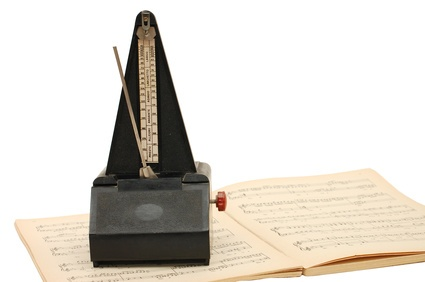# 混合型号排序–简单实例生产线上不同型号可能有不同的工作量，这会使生产线的效率降低。一个可行的解决方案是混合型号排序，这是一种调整产品生产顺序的方法，以使所有工站工作量能够平稳。在前面几篇文章中，我做了简单介绍，看如何避免这个问题，以及是否可以调整产能。但特别是对于大型复杂生产线（如汽车总装线），针对这种问题排序通常是比较合适方法。

## 关于节拍时间如果一个工站不同型号工作内容不一样，那么节拍时间也需要混合不同的型号。需要将该站的平均循环时间（cycle time）调整到与整线循环时间一样，更确切的说是节拍时间takt time而不是循环时间cycle time。更多关于Cycle time和takt time的区别，请看我的文章《衡量生产速度的不同方法》。

### 双门和四门车型实例

• TT：线节拍时间（line takt）
• TT2：两门车型节拍时间
• TT4:：四门车型节拍时间
• P2：两门车型占比
• P4：四门车型占比${ TT = TT_2 \cdot P_2 + TT_4 \cdot P_4}$${ P_2 + P_4 = 100 \%}$${ P_4 = 100 \% - P_2}$${ TT_4 = TT_2 \cdot 2}$${ TT = TT_2 \cdot P_2 + TT_2 \cdot 2 \cdot (100 \% - P_2)}$${ TT = TT_2 (P_2+ 200 \% - 2 \cdot P_2)}$${ TT = TT_2 ( 200 \% - P_2)}$${ TT_2 = \frac{TT}{200 \% - P_2}}$

### 通用公式上面的例子只针对两种型号（两门和四门），我们完全知道它们之间节拍的关系（四门需要两倍的时间）。 现实中，你会有很多不同型号，它们之间的关系可能也不是很明确。时间甚至可能在零和最大值之间变化（例如是否要安装天窗）。一般来说，如果你有k个型号，并有：

• TT：线节拍时间（line takt）
• TTn：n型号的节拍时间
• Pn：n型号的占比${ TT = \sum_{n=1}^{k} TT_n \cdot P_n }$${ \sum_{n=1}^{k} P_n = 100 \%}$

P.S.非常感谢Mark Warren的意见。

## 系列文章

Translated by Xie Xuan